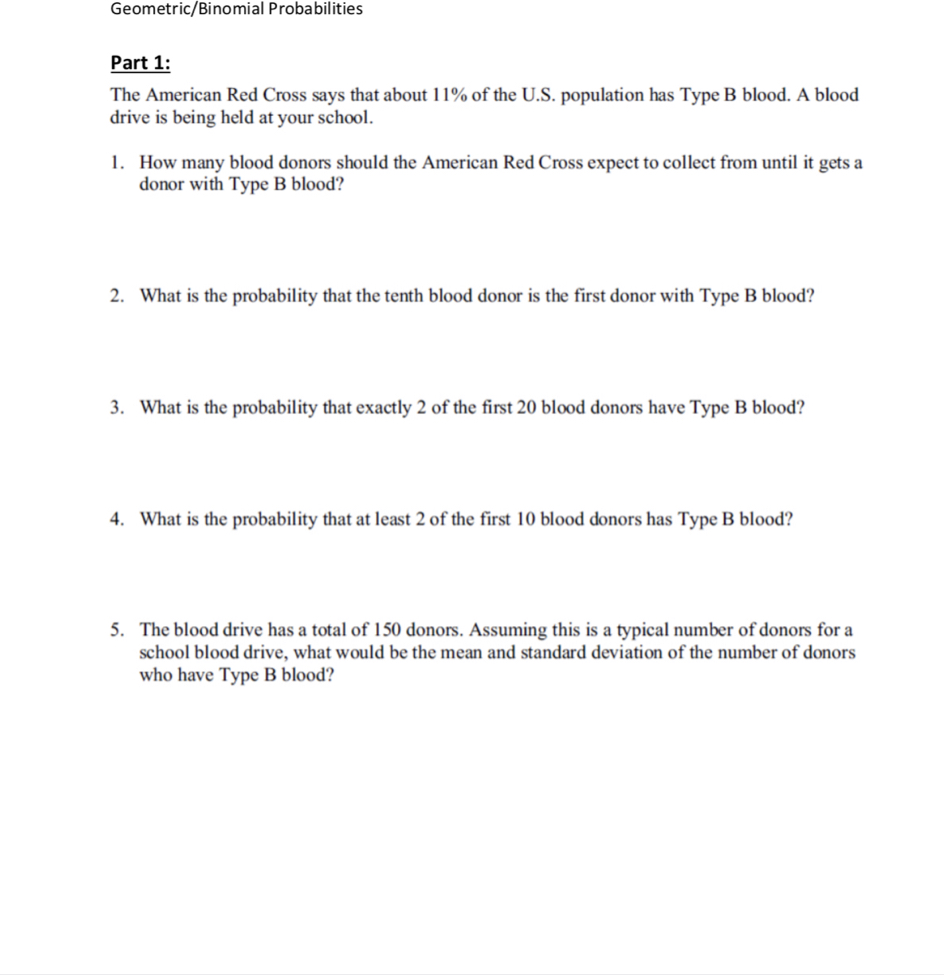# Geometric/Binomial ProbabilitiesPart 1:The American Red Cross says that about 1 190 of the US. population has Type B blood. A blooddrive is being held at your school.How many blood donors should the American Red Cross expect to collect from until it gets adonor with Type B blood?1.2. What is the probability that the tenth blood donor is the first donor with Type B blood?. What is the probability that exactly 2 of the first 20 blood donors have Type B blood?4.What is the probability that at least 2 of the first 10 blood donors has Type B blood?5. The blood drive has a total of 150 donors. Assuming this is a typical number of donors for aschool blood drive, what would be the mean and standard deviation of the number of donorswho have Type B blood?

Question
31 views

I am having trouble answering questions number 4 and 5. Thank youhelp_outlineImage TranscriptioncloseGeometric/Binomial Probabilities Part 1: The American Red Cross says that about 1 190 of the US. population has Type B blood. A blood drive is being held at your school. How many blood donors should the American Red Cross expect to collect from until it gets a donor with Type B blood? 1. 2. What is the probability that the tenth blood donor is the first donor with Type B blood? . What is the probability that exactly 2 of the first 20 blood donors have Type B blood? 4. What is the probability that at least 2 of the first 10 blood donors has Type B blood? 5. The blood drive has a total of 150 donors. Assuming this is a typical number of donors for a school blood drive, what would be the mean and standard deviation of the number of donors who have Type B blood? fullscreen
check_circle

Step 1

Introduction to binomial distribution:

Consider a random experiment involving n independent trials, such that the outcome of each trial can be classified as either a “success” or a “failure”. The numerical value “1” is assigned to each success and “0” is assigned to each failure.

Moreover, the probability of getting a success in each trial, p, remains a constant for all the n trials. Denote the probability of failure as q. As success and failure are mutually exclusive, q = 1 – p.

Let the random variable X denote the number of successes obtained from the n trials. Thus, X can take any of the values 0,1,2,…,n.

Then, the probability distribution of X is a Binomial distribution with parameters (n, p) and the probability mass function (pmf) of X, that is, of a Binomial random variable, is given as:

Step 2

4.

Obtain the probability that at least 2 of the first 10 donors have type B blood:

The size of the sample taken from the population of blood donors is n = 10. It is said that it is a random sample, imp-lying independence among each other. As a result, the 10 blood donors may be considered as 10 independent trials.

Consider the event of having a type B blood as a “success”. American Red Cross says that 11% of the U.S. population has Type B blood. Thus, the probability that a donor having type B blood, that is, the probability of success in each trial is p = 0.11. Then q = 1 – 0.11 = 0.89.

Consider X as the number of donors among the 10 sampled donors, who have the type B blood. Then, X has a Binomial distribution with parameters (n = 10, p = 0.11).

The probability that at least 2 of the first 10 donors have type B blood is obtained as 0.3028 from the calculation given below:

Step 3

Normal approximation to binomial distribution:

The pmf for the binomial distribution or the probability of obtaining x successes in n independent trails of a binomial experiment is,

P(x) = nCx * px * (1 – p)n–x

The mean and standard deviation for the binomial variate is E(x) = n * p and S.D(x) = SQRT(n *p *(1 – p)).

It is given that, about 11% of the U.S. population has Type B blood.

The probability of success (U.S. population with Type B blood) = p = 11/100 = 0.11.

The probability of failure (U.S. population not with Type B b...

### Want to see the full answer?

See Solution

#### Want to see this answer and more?

Solutions are written by subject experts who are available 24/7. Questions are typically answered within 1 hour.*

See Solution
*Response times may vary by subject and question.
Tagged in

### Other